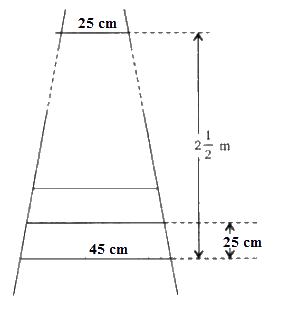# Ex.5.4 Q3 Arithmetic progressions Solutions - NCERT Maths Class 10

Go back to  'Ex.5.4'

## Question

A ladder has rungs $$25\,\rm{ cm}$$ apart. (See figure). The rungs decrease uniformly in length from $$45\,\rm{ cm}$$ at the bottom to $$25\,\rm{ cm}$$ at the top. If the top and bottom rungs are \begin{align} 2\frac{1}{2} \,\rm{m} \end{align} apart, what is the length of the wood required for the rungs?

[Hint: number of rungs = $$\frac{{250}}{{25}}$$ ]Video Solution
Arithmetic Progressions
Ex 5.4 | Question 3

## Text Solution

What is Known?

The rungs decrease uniformly in length from $$45\,\rm{ cm}$$ at the bottom to $$25\,\rm{ cm}$$ at the top and the top and bottom rungs are $$2\frac{1}{2}\,\rm{m}$$ apart.

What is Unknown?

The length of the wood required for the rungs

Reasoning:

Sum of the first $$n$$ terms of an AP is given by $${S_n} = \frac{n}{2}\left[ {a + l} \right]$$

Where $$a$$ is the first term, $$l$$ is the last term and $$n$$ is the number of terms.

Steps:

Given:

• Distance between the rungs $$= 25\,\rm{cm}$$
• Distance between the top and bottom rungs \begin{align}=2\frac{1}{2} \;\rm{m} = 2\frac{1}{2} \times100 \,\rm{cm}\end{align}

$$\therefore$$ Total number of rungs

\begin{align}&= \frac{{2\frac{1}{2} \times 100}}{{25}} + 1\\&= \frac{{250}}{{25}} + 1 \\&= 11\end{align}

From the given Figure, we can observe that the lengths of the rungs decrease uniformly, hence we can conclude that they will be in an AP

The length of the wood required for the rungs equals the sum of all the terms of this A.P.

• First term, $$a = 45$$
• Last term, $$l = 25$$
• Number of terms, $$n = 11$$

Hence Sum of $$n$$ terms of the AP Series,

\begin{align}{S_n} &= \frac{n}{2}\left[ {a + l} \right]\\{S_{11}} &= \frac{{11}}{2}\left[ {45 + 25} \right]\\ &= \frac{{11}}{2} \times 70\\&= 11 \times 35\\&= 385\end{align}

Therefore, the length of the wood required for the rungs is $$385 \,\rm{cm.}$$

Learn from the best math teachers and top your exams

• Live one on one classroom and doubt clearing
• Practice worksheets in and after class for conceptual clarity
• Personalized curriculum to keep up with school